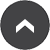# Year 4 - Mr Dawes

## Autumn Term Maths Overview

29 Sep 2016

Dear Parents and Carers,

Here is a copy of the Autumn term Maths overview and the topics we will be covering.

 Autumn Term 1 Wk Strands Weekly Summary 1 Mental addition and subtraction (MAS); Problem solving, reasoning and algebra (PRA) Finding pairs with a total of 100; adding to the next multiple of 100 and subtracting to the previous multiple of 100; subtract by counting up to find a difference; adding several numbers 2 Number and place value (NPV); Mental addition and subtraction (MAS) Read, write 4-digit numbers and know what each digit represents; compare 4-digit numbers using < and > and place on a number line; add 2-digit numbers mentally; subtract 2-digit and 3-digit numbers 3 Mental multiplication and division (MMD); Problem solving, reasoning and algebra (PRA); Written multiplication and division (WMD); Fractions, ratio and proportion (FRP) Learn × and ÷ facts for the 6 and 9 times-table and identify patterns; multiply multiples of 10 by single-digit numbers; multiply 2-digit numbers by single-digit numbers (the grid method); find fractions of amounts 4 Measurement (MEA); Decimals, percentages and their equivalence to fractions (DPE) Tell and write the time to the minute on analogue and digital clocks; calculate time intervals; measure in metres, centimetres and millimetres; convert lengths between units; record using decimal notation 5 Written addition and subtraction (WAS) Add two 3-digit numbers using column addition; subtract a 3-digit number from a 3-digit number using an expanded column method (decomposing only in one column)

 Autumn Term 2 Wk Strands Weekly Summary 6 Mental multiplication and division (MMD); Problem solving, reasoning and algebra (PRA); Fractions, ratio and proportion (FRP) Double 3-digit numbers and halve even 3-digit numbers; revise unit fractions; identify equivalent fractions; reduce a fraction to its simplest form; count in fractions (each fraction in its simplest form) 7 Decimals, percentages and their equivalence to fractions (DPE); Number and place value (NPV); Written addition and subtraction (WAS); Mental addition and subtraction (MAS) Look at place value in decimals and the relationship between tenths and decimals; add two 4-digit numbers; practise written and mental addition methods; use vertical addition to investigate patterns 8 Decimals, percentages and their equivalence to fractions (DPE); Measurement (MEA); Statistics (STA); Problem solving, reasoning and algebra (PRA) Convert multiples of 100 g into kilograms; convert multiples of 100 ml into litres; read scales to the nearest 100 ml; estimate capacities; draw bar charts, record and interpret information 9 Number and place value (NPV); Written addition and subtraction (WAS); Mental addition and subtraction (MAS) Round 4-digit numbers to the nearest: 10, 100 and 1000; subtract 3-digit numbers using the expanded written version and the counting up mental strategy and decide which to use 10 Mental multiplication and division (MMD); Written multiplication and division (WMD); Problem solving, reasoning and algebra (PRA) Use the grid method to multiply 3-digit by single-digit numbers and introduce the vertical algorithm; begin to estimate products; divide numbers (up to 2 digits) by single-digit numbers with no remainder, then with a remainder

Go to the page-top